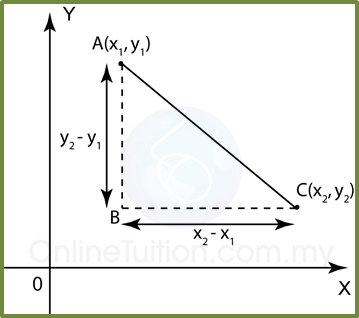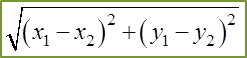\

# 7.1 Distance between Two Points

7.1 Distance between Two Points
(x1, y1) and (x2, y2) are two points on a coordinate plane as shown below. BC is parallel to the x-axis and AB is parallel to the y-axis.  Hence  ∆ ABC = 90°.Distance between Point A and C =Example:
Find the distance between the points P (2, 2) and Q (–4, –5).

Solution:
Let P (2, 2) = (x1, y1 ) and Q (–4, –5) = (x2, y2 ).